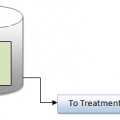## Measurement of Sediment Oxygen Demand (SOD)

Measurement of Sediment oxygen demand (SOD) is a measure of the oxygen demand characteristics of the bottom sediment which affects the dissolved oxygen resources of the overlying water. To measure SOD, a bottom sample is especially designed to entrap and seal a known quantity of water at the river bottom. Changes in DO concentrations (approximately 2 h, or until the DO usage curve is clearly defined) in the entrapped water are recorded by a DO probe fastened in the sampler.

The test temperature should be recorded and factored for temperature correction at a specific temperature.SOD curves can be plotted showing the accumulated DO used (y-axis) versus elapsed time (x-axis). SOD curves resemble first-order carbonaceous BOD curves to a great extent; however, first order
kinetics are not applicable to the Upper Illinois Waterway’s data. For the most part, SOD is caused by bacteria reaching an “unlimited” food supply.

Consequently, the oxidation rates are linear in nature. The SOD rates, as taken from SOD curves, are in linear units of milligrams per liter per minute (mg/(L . min)) and can be converted into grams per square meter per day (g/(m2 . d)) for practical application. A bottle sampler SOD rate can be formulated as:

SOD =1440 SV /103 A

where SOD = sediment oxygen demand, g/(m2 . d)
S = slope of stabilized portion of the curve, mg/(L . min)
V = volume of sampler, L
A = bottom area of sampler, m2## Author:T.P.Sivanandan

#### Why Fine Bubbles are Better than Coarse bubble in Aeration Tank#### How to Calculate Lime Dosage Requirement in Sedimentation Process